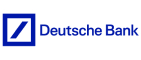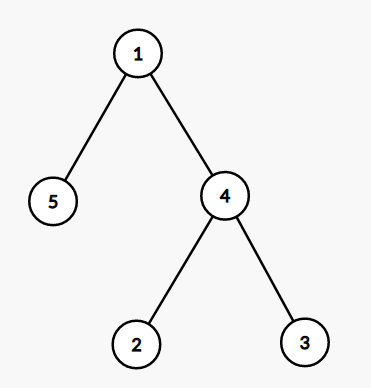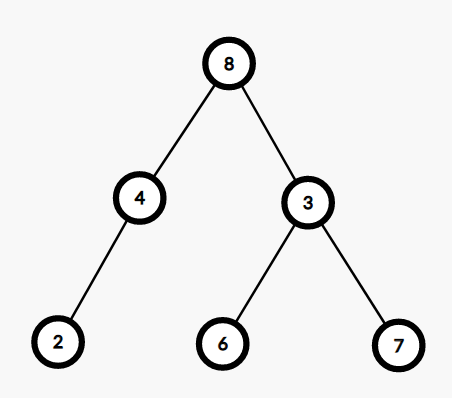New update is available. Click here to update.
Topics

# Pairs Violating BST

Moderate0/80
Average time to solve is 25m
0 upvote## Problem statement

You are given a binary tree and you need to find the number of pairs violating BST property.

BST tree has the following properties:-

``````1. For every node, it is greater than or equal to its left child and less than or equal to its right child.
2. Node is greater than equal to the maximum value of its left subtree and less than the minimum value of its right subtree.
3. The maximum in the left sub-tree must be less than equal to the minimum in the right subtree.
``````
For example in the following tree:-``````The numbers of pairs violating BST property are:-

(1,5) : 1 should be greater than its left child value.

(4,3) : 4 should be less than its right child value.

(5,4),(5,3):  Maximum of left subtree of 1 is 5 greater
than 2 and 3 of its right subtree.
``````
Detailed explanation ( Input/output format, Notes, Images )
Constraints
``````1 <= 'T' <= 10
1 <= Number of nodes in Tree <= 5000
1 <= Value of nodes <= 10 ^ 5

Where 'T' is the number of test cases.

Time Limit: 1 sec.
``````
Sample Input 1:
``````1
8 4 3 2 -1 6 7 -1 -1 -1 -1 -1 -1
``````
Sample Output 1:
``````5
``````
Sample Output Explanation
`````` For the above input tree is:-
````````````Thus pairs violating BST property are:-
(8,3) :- Greater than its right child.
(8,6) :- Greater than node on its right side.
(8,7) :- Greater than node on its right side.
(3,6):- The value of 3 is not more than its left child.
(4,3):- The value in the left subtree is greater than the
value of the right subtree.

Thus the number of pairs is 5.
``````
Sample Input 2:
``````2
35 44 26 -1 50 37 20 -1 -1 43 36 -1 -1 -1 -1 -1 -1
49 48 26 32 36 40 31 30 -1 25 40 -1 -1 -1 40 -1 -1 -1 -1 -1 -1 -1 -1
``````
Sample Output 2:
``````24
25
``````Console